# Using Bootstrap in React

In this lesson we will learn how to use a Bootstrap grid in React.

Disclaimer: I will explain only the key concepts and basic usage. If you wise to master the use of Bootstrap I strongly suggest you finish this course first and come back here. On the other hand, if you are familiar with Bootstrap you can scan this lesson to check how to use it along with React or directly jump to the next lesson.

## 1. The concept of a grid

Currently, our app has two equal columns which are placed next to each other on a large screen. As soon as we resize our browser window they nest one below the other: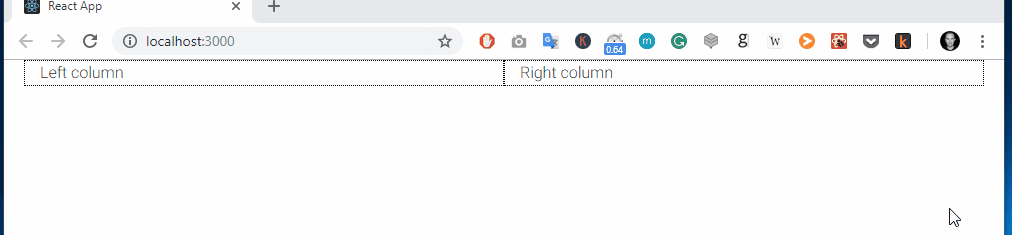What is the Bootstrap Grid?
In nutshell, it's a mechanism which allows us to create a responsive website. The Bootstrap grid allows us to define rows, and each row consists of up to 12 columns.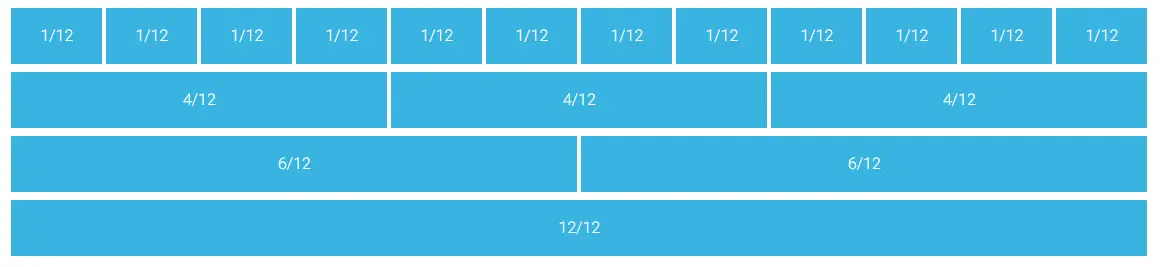You can either use one column that will be 12 blocks long or use multiple smaller blocks. The only rule is that you cannot exceed more than 12 blocks in a row. Blocks don't have to be equal: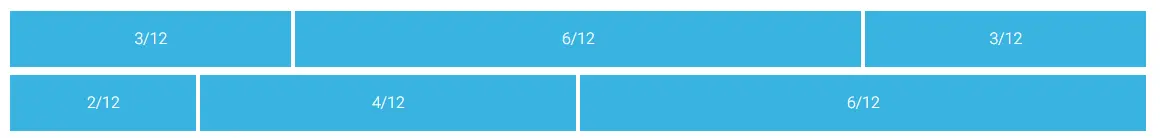And you can skip some of the columns, as well as align them horizontally: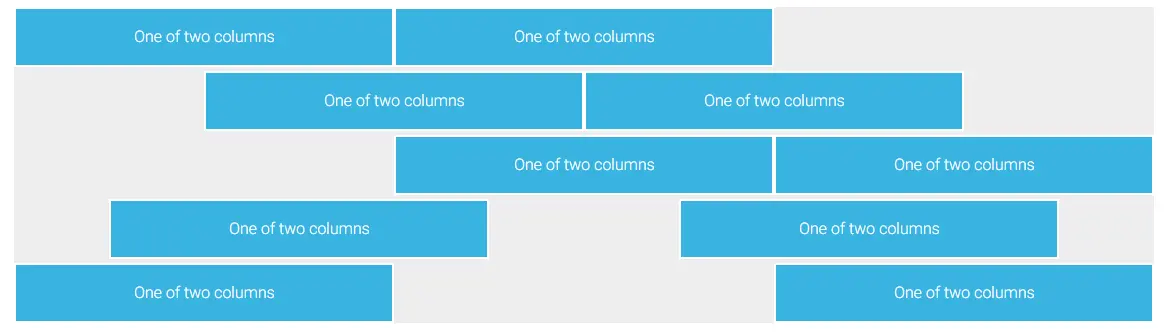For more advanced usage you can check our docs:

## 2. Responsive breakpoints

Bootstrap is developed to be mobile—first, so it uses a handful of media queries to create sensible breakpoints for our layouts. These breakpoints are mostly based on minimum viewport widths and allow you to scale up elements as the viewport changes.

This is one of the most important features — in other words: you can define a different column size for different screen sizes.  Bootstrap lists 5 different screen sizes, extra small (default), small (sm), medium (md), large (lg)  and extra large (xl).  Each size has a breaking point defined according to the table below: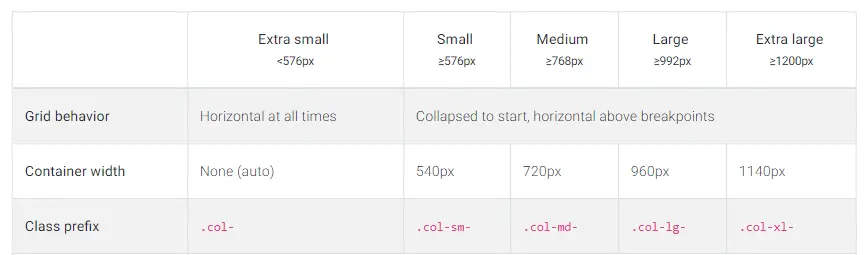Bootstrap responsive breakpoints table

Coming to the conclusion — this super handy tool allows us to create content which will automatically adjust to the screen size. Let's try the following code:
``````
import React, { Component } from "react";
import ReactDOM from "react-dom";
import '@fortawesome/fontawesome-free/css/all.min.css';
import "bootstrap-css-only/css/bootstrap.min.css";
import "mdbreact/dist/css/mdb.css";
import { MDBContainer, MDBRow, MDBCol } from "mdbreact";
import "./index.css";
class App extends Component {
state = {};
render() {
return (
<React.Fragment>
<MDBContainer>
<MDBRow>
<MDBCol xl="1" lg="2" md="4" sm="6" size="12">xl=1 lg=2 md=4 sm=6 xs=12</MDBCol>
<MDBCol xl="1" lg="2" md="4" sm="6" size="12">xl=1 lg=2 md=4 sm=6 xs=12</MDBCol>
<MDBCol xl="1" lg="2" md="4" sm="6" size="12">xl=1 lg=2 md=4 sm=6 xs=12</MDBCol>
<MDBCol xl="1" lg="2" md="4" sm="6" size="12">xl=1 lg=2 md=4 sm=6 xs=12</MDBCol>
<MDBCol xl="1" lg="2" md="4" sm="6" size="12">xl=1 lg=2 md=4 sm=6 xs=12</MDBCol>
<MDBCol xl="1" lg="2" md="4" sm="6" size="12">xl=1 lg=2 md=4 sm=6 xs=12</MDBCol>
<MDBCol xl="1" lg="2" md="4" sm="6" size="12">xl=1 lg=2 md=4 sm=6 xs=12</MDBCol>
<MDBCol xl="1" lg="2" md="4" sm="6" size="12">xl=1 lg=2 md=4 sm=6 xs=12</MDBCol>
<MDBCol xl="1" lg="2" md="4" sm="6" size="12">xl=1 lg=2 md=4 sm=6 xs=12</MDBCol>
<MDBCol xl="1" lg="2" md="4" sm="6" size="12">xl=1 lg=2 md=4 sm=6 xs=12</MDBCol>
<MDBCol xl="1" lg="2" md="4" sm="6" size="12">xl=1 lg=2 md=4 sm=6 xs=12</MDBCol>
<MDBCol xl="1" lg="2" md="4" sm="6" size="12">xl=1 lg=2 md=4 sm=6 xs=12</MDBCol>
</MDBRow>
</MDBContainer>
</React.Fragment>
);
}
}
ReactDOM.render(<App />, document.getElementById("root"));

``````

Run the app and resize the browser size: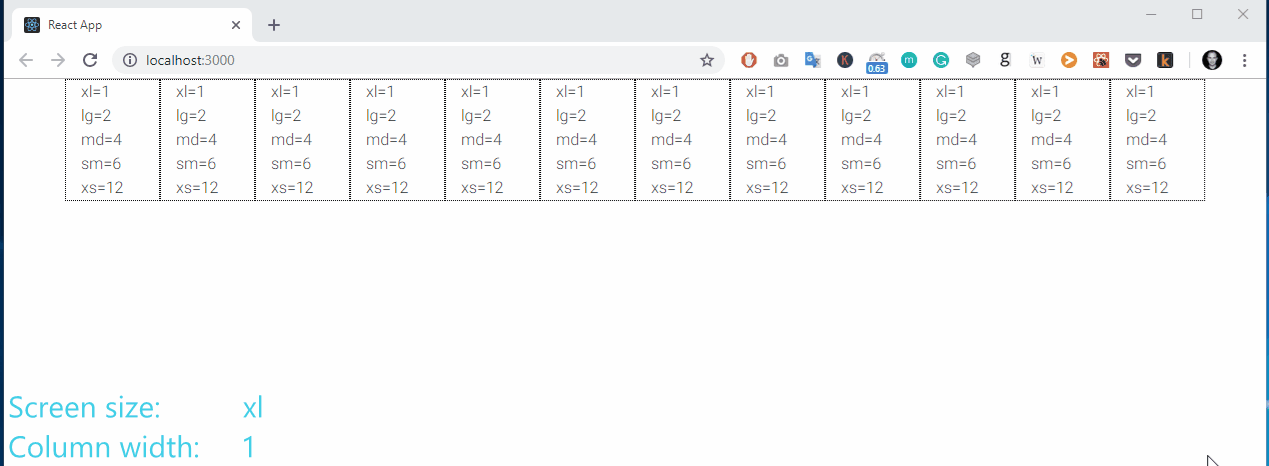I hope that you now you can understand how easy it is to build responsive components using Bootstrap. Let's get back to our app. We want to create a two—column layout which will be visible on the desktop (extra large and large) as well as tablets in horizontal aspect (medium). For smaller screen sizes we want our columns to nest one below the other.

## 4. The responsive grid of our Calendar App

Replace the render() function template with the following code:

``````
<MDBContainer>
<MDBRow>
<MDBCol md="9">Left column</MDBCol>
<MDBCol md="3">Right column </MDBCol>
</MDBRow>
</MDBContainer>
``````
This will render the expected result. Note that we have provided only a medium breaking point. Bootstrap by default assumes that we want 9/3 ratio not only for a medium screen but also for bigger screens, as well as that we want everything which is lower than md to display on full 12 width.